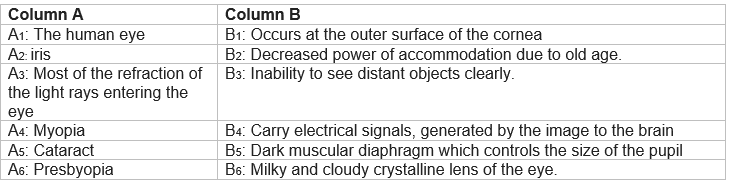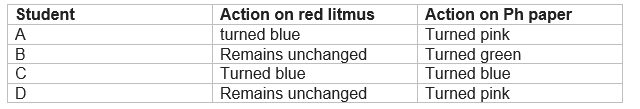# Class 10 Science Half Yearly Sample Paper -3

In this page we have Class 10 Science Half Yearly Sample Paper -3 . Hope you like them and do not forget to like , social share and comment at the end of the page.

## Section A

Question 1.
Give the meaning of power of accommodation of the normal eye.

Question 2.
Write the names of these functional groups.
—C= C—, C= C, —C1, —OH

Question 3.
Give one difference between fossil fuels and the sun as direct source of energy.

Question 4.
(a) How does the atomic structure (electronic arrangement ) change in a period with an increase in atomic number from left to right?
(b) How does the valency of elements change on moving from left to right in the second period of the periodic table?

Question 5.
What are connecting links? How do they provide us information about the evolution of an organism?

Question 6.
Compare the radii of two species X and Y. Give reasons for your answer. (i) X has 12 protons and 12 electrons.
(ii) Y has 12 protons and 10 electrons.

Question 7.
A convex mirror used for rear-view in an automobile, has radius of curvature of 3m. If a bus is located at 5m from this mirror, find the position, nature and size of the image.

Question 8.
A concave lens of focal length 15 cm forms and image 10 cm from the lens. How far is the object placed from the lens? Draw the ray diagram

Question 9.
A few defects of the human eye and the functions/relevant definition, information about different parts of the human eye are listed in columns A and B below; Select the pairs, in the two columns, that match them.Question 10.
(a) What are transition elements?
(b) Which amongst the following are transition elements?
K, Mn, Ca, Cr, Cu, Cs, He, Ar and Pt
and why?

Question 11.
Mrs. Anita observed that her cooking utensils are becoming black in colour and the flame of her gas stove is yellowish in colour. Her neighbor Mrs. Anand when came to her, told the reason why her utensils become black and advised her to clean the stove or gas burner time to time.
Answer the following questions on the basis of the above text:
(a) What can be the reason for this sooty flame?
(b) What steps should be taken to stop this process?
(c) What is the associated value that the learners will get from this passage?

Question 12.
A glass prism is able to produce a spectrum, when white light passes through it but a glass slab does not produce any spectrum. Explain why is itso?

Question 13.
In the context of conservation of natural resources, explain the terms reduce, recycle and reuse. Identify two materials for each category from the material that we use in daily life.
Question 14.
What is puberty? Name any two changes seen in girls at the time of puberty.

Question 15.
(a) What is vegetative propagation?
(b) What methods will you use for growing jasmine and rose plants?

Question 16.
Explain the two kinds of variations. Which of the two is heritable?

Question 17.
Can sexual reproduction lead to the variations in characters, speciation and evolution? Explain.

Question 18.
Give the basic feature of mechanism of inheritance.

Question 19.
Using ray diagram, explain the laws of formation of image by divergent lens.
or
(a) Draw a ray diagram showing formation of image by convergent lens, when object is placed between lens and focus.
(b) State the nature of the image formed.
(c) Will the image position and the focal length, be positive or negative?
(d) Give one application of this lens for this position of the object.

Question 20.
(a) Why are the small numbers of surviving tigers a cause of worry from genetic point of view?
(b) Does the occurrence of diversity of animals on earth suggest their diverse ancestry? Discuss this in the light of evolution.
or
(a) Explain the process of evolution in pterosaur.
(b) Describe the evolution of eye in flatworm.

Question 21.
Describe the evolution of eye in flatworm.
or
Describe the process of double fertilization with the help of a diagram.

Question 22.
(a) Write the name and molecular formula of an organic compound having its name suffixed with-ol and having two carbon atoms in the molecule. With the help of a balanced chemical equation, indicate what happens when it is heated with excess of cons. H2SO4?
(b) What is substitution reaction? Give an example.
Or
(a) What is observed?
(i) When? 2 mL of ethanol and 2Ml of glacial acetic acid are taken in a test tube along with a few drops of concentrated sulphuric acid?
(ii) The mixture is warmed in a water bath for five minutes and the contents are poured into another beaker containing about 30-40 mL of water?
(b) Name the process by which an ester can be converted back to alcohol and sodium salt of carboxylic acid.
(c) Give reasons
(i) Carbon cannot form C4+ /C4- ions.
(ii) Unlike ethanol intake of methanol in very small quantity can cause death.
(iii) Why only unsaturated hydrocarbons undergo addition reactions and not the saturated hydrocarbons?

Question 23.
An element is place in 2nd group and 3rd period of the periodic table, burns in the presence of oxygen to for a basic oxide.
(a) Identify the element.
(b) Write its electronic configuration.
(c) Write the balanced equation, when it burns in the presence of air.
(d) Write the balanced equation, when this oxide is dissolved in water.
(e) Draw the electron dot structure for the formation of this oxide. or
Properties of the elements are given below.
Where would you locate the following elements in the periodic table?
(a) A soft metal stored under kerosene.
(b) An element with variable (more than one) valence stored under water.
(c) An element which is tetravalent and forms the basis of organic chemistry.
(d) An element which is an inert gas with atomic number
(e) An element whose thin oxide layer is used to make other elements corrosion resistant by the process of anodizing.

Question 24.
Explain the refraction of light through a triangular glass prism using a labeled ray diagram. Hence, define the angle of deviation.

## Section-B

Question 25.
Some steps not in proper order are given below to determine the focal length of a convex lens to obtain a sharp image of a distant object.
A- Measure the distance between the lens and screen.
B- Adjust the position of the lens to form a sharp image.
C- Selected a suitable distant object.
D- Hold the lens between the object and the screen with its face parallel to the screen.
(a) C, A, D, B
(b) C, D, B, A
(c) C, A, B,D
(d) A,B,C,D

Question 26.
Which of the following vegetables are examples of homologous structure?
(a) Potato and tomato
(c) Carrot and tomato

Question 27.
2 mL of ethnic acid was taken in each, test tube I and test tube II. A red litmus paper was introduced in test tube I and a PH paper were introduced in test tube II. The experiment was performed by four students A, B, C, D and they reported their observations as given in the table.The correct set of observations were made by student
(a) A
(b) B
(c) C
(d) D

Question 28.
If Ph paper is dipped in acetic acid, the colour of the strip would be
(a) Green
(b) blue
(c) orange
(d) black

Question 29.
Draw the ray diagrams to show that a ray parallel to the principal axis, after reflection, will pass through the principal focus in case of a concave mirror and appear to diverge from the principal focus in case of a convex mirror.

Question 30.
What is Tnydall effect?

Question 31.
What are the harmful effects of the negligence of the local knowledge and local need in the forest management particles?

## Summary

This Class 10 Science Half Yearly Sample Paper -3 for TERM 1 is prepared keeping in mind the latest syllabus of CBSE . This has been designed in a way to improve the academic performance of the students. If you find mistakes , please do provide the feedback on the mail.